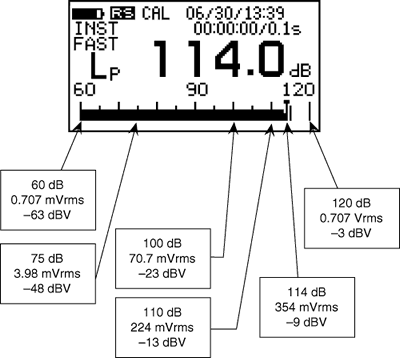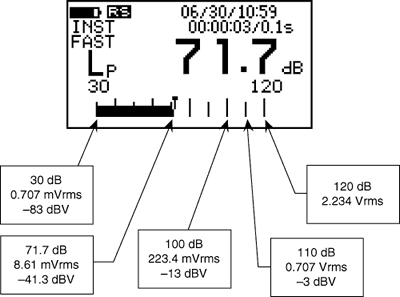Sound Level Meter FAQAC output1. AC output in normal range

The specifications of AC output are as follows.

• Full scale output level 0.707 Vrms±15%

• Offset voltage ±10 mV or less

Therefore, when a steady sine wave is input, the AC output at the upper limit for the bar indicator is;

±1 V = 0.707 Vrms = -3 dBV

When the measurement level of the steady signal is adB above the upper limit of the bar indicator, the output is expressed as follows;

0.707×10a/20 Vrms = -3+a dBV

For example, when the measurement level of the steady signal is -20 dB above the upper limit of the bar indicator, the output is expressed as follows; (When the level range is 60 to 120 dB, the measurement level is equivalent to 100 dB. When the level range is 40 to 120 dB in real-time mode, it is equivalent to 100 dB.)

0.70×10(-20)/20 = 70.7 mVrms

-3+(-20) = -23 dBV

As the electrical calibration signal is -6 dB above the upper limit of the bar indicator, the AC output is 354 mVrms or -9 dBV. (When the level range is 60 to 120 dB, the measurement level is equivalent to 114 dB.) When the AC output level is bVrms or adBV with steady signal input, the indicated value is separated from the upper limit of the bar indicator by;

20×Log10(b/0.707) dB or a+3 dB

For example, when the AC output is 100 mVrms = -20 dBV, the indicated value is separated from the full scale by;

20×Log10(0.1/0.707) = -17 dB

-20+3 = -17 dB

When the level range is 60 to 120 dB, the measurement level is equivalent to 103 dB. When the level range is 40 to 120 dB in real-time mode, it is equivalent to 103 dB. The maximum AC output level is ±1.00 V and linearity is guaranteed for up to this level. The maximum value of the sine wave indicating 10 dB greater than the full scale is ±3.16 V. If the AC output level exceeds this value, the A/D converter overflows and the over mark is displayed. With the LA-5110 and LA-2110, measurement is invalid if sound pressure exceeding 140 dB is input. With the LA-5120, measurement is invalid if sound pressure exceeding 130 dB is input.2. AC output in wide range

The specifications of AC output are as follows;

• Full scale output level 2.234 Vrms ±15%

• Offset voltage ±10 mV or less

Therefore, when a steady sine wave is input, the AC output at the upper limit for the bar indicator is;

±3.16 V = 2.234 Vrms = +7 dBV

When the measurement level of the steady signal is adB above the upper limit of the bar indicator, the output is expressed as follows;

2.234×10a/20 Vrms = 7+a dBV

For example, when the measurement level of the steady signal is -20 dB above the upper limit of the bar indicator, the output is expressed as follows;

2.234×10(-20)/20 = 223.4 mVrms

7+(-20) = -13 dBV

As the electrical calibration signal is -16 dB above the upper limit of the bar indicator, the AC output is 354 mVrms or -9 dBV. (When the level range is 30 to 120 dB, the measurement level is equivalent to 104 dB.) When the AC output level is bVrms or adBV with steady signal input, the indicated value is separated from the upper limit of the bar indicator by;

20×Log10(b/2.234) dB or a-7 dB

For example, when the AC output is 8.61 mVrms = -41.3 dBV, the indicated value is separated from the full scale by;

20×Log10(0.00861/2.234) = -48.3 dB

-41.3-7 = -48.3 dB

When the level range is 30 to 120 dB, the measurement level is equivalent to 71.7 dB. The maximum AC output level is ±3.16 V and the maximum value of the sine wave indicating 10 dB greater than the full scale is ±10.0 V. In wide range, the AC output level at the lower limit of the linearity range is only 70 μV. Therefore, to cover the entire linearity range, recorders and analyzers with low noise level are required.Revised:2001.10.12• Copyright © ONO SOKKI CO.,LTD All Rights Reserved.